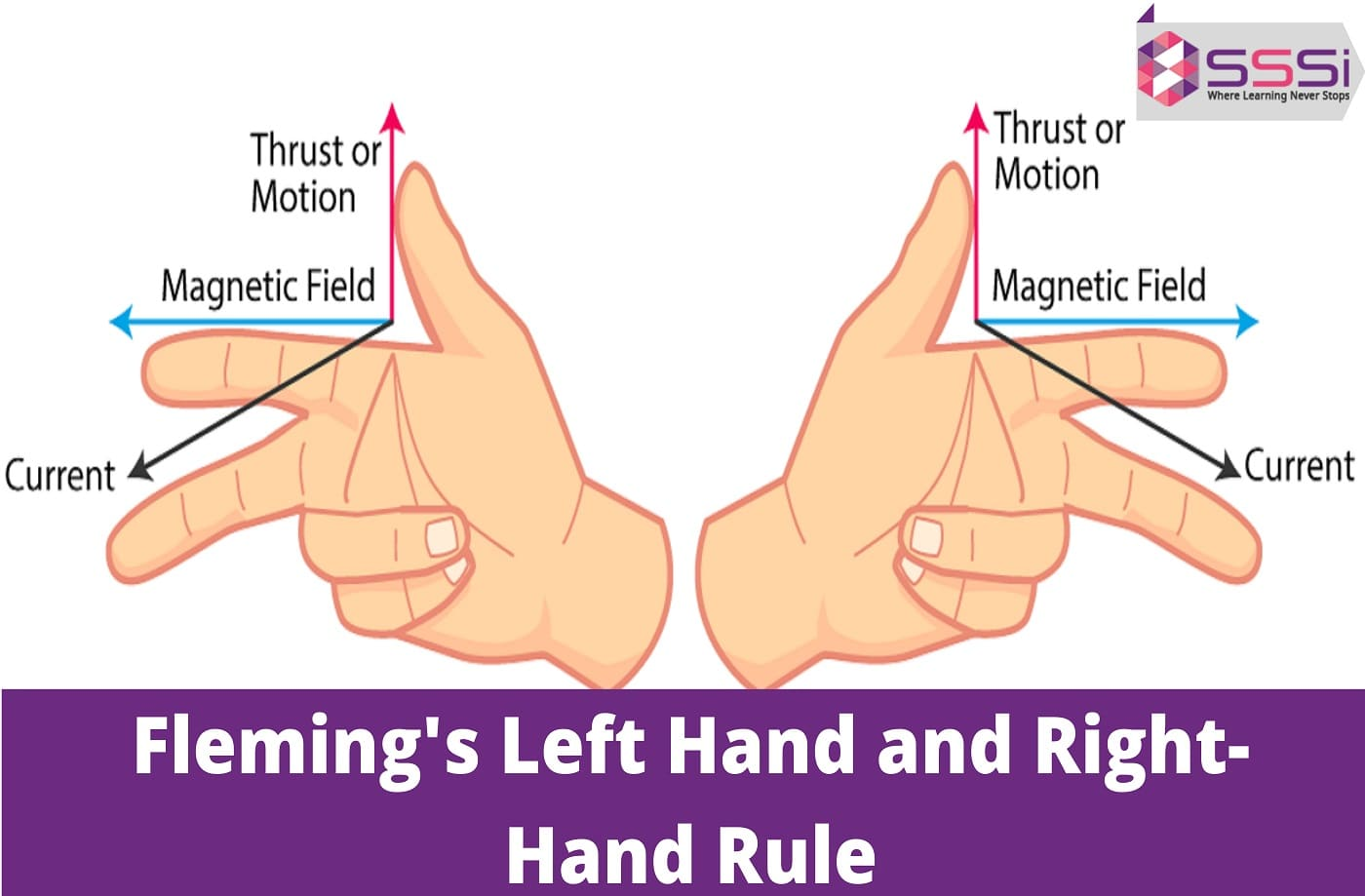# What is Fleming's Left Hand and Right-Hand Rule?General
June 07, 2023

Did you know that in a magnetic field current-carrying conductor will encounter force? It is called electromagnetic force or emf. All contemporary electric motors and generators are built around this feature. So we know there's a force acting on the current-carrying conductor, but how do we know which way it's pulling? If only a simple procedure could be used in practically every circumstance. Well, Fleming's left-hand rule will do that.

Similarly, when a conductor is energized in a magnetic field, an induced current will be present in the conductor. By using Fleming's right-hand rule, power direction can be easily obtained.

Therefore, there is a connection between the magnetic field, and the force, and still in both laws. These rules do not indicate the size but indicate the direction of any of these parameters where the direction of the other two boundaries is known.

In the case of electric motors, Fleming's Left-handed rule applies, while Fleming's Right-handed rule applies to electric generators.

## Understand Fleming’s Left Hand Rule

A force is exerted on the current-carrying conductor in a direction perpendicular to the direction of the magnetic field and current whenever it is set inside the magnetic field. It is mainly suitable to find the direction of the force.

It is stated as follows:

"Your left hand's forefinger, middle finger, and thumb should all be at a right angle (90degree) to one another the forefinger, middle finger, and thumb will all point in the same direction"

• Forefinger points in the direction of the magnetic field
• Middle finger points in the current direction
• Thumb points in the direction of force

## Understand Fleming’s Right-Hand Rule

Faraday's law for the introduction of electromagnetic induction states that whenever a conductor is placed inside a magnetic field, the current induction will operate on it. There will be a relationship between the direction of the applied force, the magnetic field, and the current if the conductor is pushed firmly into the magnetic field. Fleming's right-hand rule determines the relationship between these three approaches.

It's stated as:

"Right finger, middle finger, and thumb should be right at each other. The middle finger will point toward the emf (or current) if the front finger points to the magnetic field and the thumb points the direction of conductor movement."

## Know the Difference Between Fleming’s Left Hand and Right-Hand Rule

 Fleming’s Left Hand Rule Fleming’s Right Hand Rule It applies to electric motors It applies to electric generators Drive to find the magenta force direction in an electric motor. Find the induced force exerting in the electric generator. Current direction is indicated by the middle finger Induced current direction is indicated by the middle finger

We have learned the basics of Fleming's left hand and right-hand rules. Let's now discuss a few questions related to this topic.

## Concept-Based Questions

Question 1: If the electron is moving up and down in the south direction by the same magnetic field, find the direction of the magnetic field.

Solution: Fleming's left-hand rule is used to determine the direction of the electron magnetic field.

The electron has a negative charge, and when it goes upwards, the current direction goes downwards. As per the question, the electron magnetic field faces south. Thus, the east is the direction of the magnetic field.

Question 2: Suppose 5A is the current flowing in the conductor, 4m is the length of the rod, and the magnetic field is generated by 3 T. Find the force produced.

Solution:

As per the question given,

I = 5A, L = 4m and B = 3 T

F = I x L x B

= 5 x 4 x 3

F = 60 N

60 N is the force produced.

If you want to learn more about its working on electric motors and generators, join our online physics classes and understand the concept from scratch. The following are the additional key benefits of our services:

• Experienced and certified tutors.
• One-on-one mentorship programme.
• Live practice questions and mock tests.
• Unlimited doubt clearing sessions and revision classes.

## Choose Modern Classes And Stay Ahead